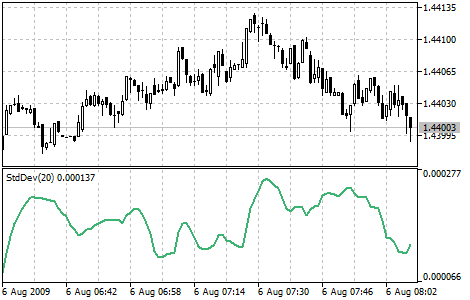# 标准方差

• 如果值太低，例如，市场非常不活跃，所以期望不久就出现峰值；
• 相反，如果太高，则意味着活跃程度不久就会降温。## 计算

StdDev (i) = SQRT (AMOUNT (j = i - N, i) / N)

AMOUNT (j = i - N, i) = SUM ((ApPRICE (j) - MA (ApPRICE , N, i)) ^ 2)

StdDev (i) ― 当前柱的标准离差;
SQRT ― 平方根;
AMOUNT(j = i - N, i) ― 从 j = i - N 到 i的平方总和;
N ― 平滑周期;
ApPRICE (j) ― j柱的获得价格;
MA (ApPRICE , N, i) ― 对于N 周期当前柱的移动平均数;
ApPRICE (i) ― 当前柱的获得价位。